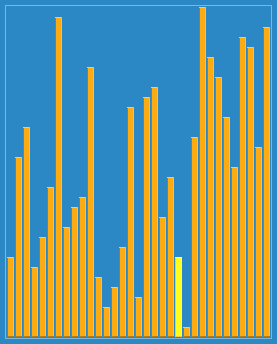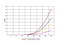# Shell Sort Algorithm

## Design and Analysis of Algorithms

Donald L. Shell introduced the Shell Sort Algorithm in the year 1959. He improvised the insertion sort algorithm that is why shell sort is also called as “diminishing Insertion sort”. It starts comparison of elements that are separated by a particular distance, the distance decreases on each iteration or pass. The distance or spacing between elements is called “interval” or “gap”.

Shell sort is very efficient in sorting the elements that are widely placed in an array compared to Insertion sort . Insertion sort takes an element and one by one compares & shifts whereas in shell sort it reduces the number of comparisons by dividing main array into sub arrays and comparing them. The interval of sub arrays is reduced at each iteration until it becomes ‘1’ .

The interval could be calculated using:

1. Original increment proposed by Donald Shell : N/2 , N/4 , …, 1.
2. Knuth increment : It uses (3^k -1)/2 as formula.
3. Hibbard’s increment: It is 1, 3, ….,(2^k -1).
4. Pratts increment: It includes number of the form 2^P*3^Q .
5. Stasevich & Papernov increment.

The Algorithm used has following steps:

1. Initialize the value of interval or gap by choosing from different increments.
2. Split the list into sub list of equal intervals.
3. Sort the sub lists.
4. Repeat until whole list is sorted or interval gets smaller than 1.

# Let us take an example to understand:Visualized representation of sorting using Shell Sort algorithm

Lets assume we have initial array to be:

[7,4,9,2,6,3] and Shell’s increment to calculate the gap .

Gap=(6/2)=3.

Step 1: [7,4,9,2,6,3] -> [2,4,9,7,6,3] (Interval/Gap:3)
Here, we take 7 and 2 & compare them. Since 2<7, they are swapped.

Step 2: [2,4,9,7,6,3] -> Already sorted (Interval/Gap:3)
Here, we take 4 and 6 & compare them. Since 6>3, they don’t swap.

Step 3: [2,4,9,7,6,3] -> [2,4,3,7,6,9] (Interval/Gap:3)
Here, we take 9 and 3 & compare them. Since 3<9, they are swapped.

Step 4: [2,4,3,7,6,9] -> Already sorted (Interval/Gap:2)
Here, we take 2, 3 and 6 & compare them. Not swapped .

Step 5: [2,4,3,7,6,9] -> Already sorted (Interval/Gap:2)
Here, we take 4, 7 and 9 & compare them. Not swapped.

Step 6: [2,4,3,7,6,9] -> Already sorted (Interval/Gap:1)
Here, we take 2 and 4 & compare them. Since 2<4, they don’t swap.

Step 7: [2,4,3,7,6,9] -> [2,3,4,7,6,9] (Interval/Gap:1)
Here, we take 4 and 3 & compare them. Since 3<4, they are swapped.

Step 8: [2,3,4,7,6,9] -> [2,3,4,6,7,9] (Interval/Gap: 1)
Here, we take 7 and 6 & compare them. Since 6<7, they are swapped.

Step 9: [2,3,4,6,7,9] -> Already sorted (Interval/Gap: 1)
Here, we take 7 and 9 & compare them. Since 7<9, they don’t swap.

The time complexity will be dependent on the Interval sequence we choose.

# Pseudocode

`procedure ShellSort()   arr: Array    /*calculate gap*/   while gap< arr.length/3 do:      gap=gap*3 + 1        end while      while gap> 0 do:      for outside=gap;outside< arr.length;outside ++ do:      /*choosing value to be inserted */      valueToInsert = arr[outside]      inside= outside;         /*shift element to right*/         while inside >gap-1 && arr[inside-gap]>= valueToInsert do:            arr[inside]=arr[inside-interval]            inside = inside-gap         end while      /*inserting num at hole position*/      arr[inside] = valueToInsert      end for   /* calculate gap*/   gap=(gap-1)/3;      end whileend procedure`

# Program for shell sort

`void ShellSort(int a[],int n){int interval = n/2;while(interval>0){for(int i=interval;i<n;i++){int temp = a[i];int j=i;while(j>=interval && a[j-interval]>temp){a[j] = a[j-interval];j =j- interval;}a[j] = temp;}interval = interval/2;}}`

# Stability of Shell Sort:

Shell sort is not stable. It is unstable because the increment based sort of elements moves it over a long distance without examining the intermediate elements. This changes the order of similar elements in the sorted list.

For example:

We have an array: [4,6,7,5,4,2,3].
The output could be given by:
[2,3,4,4,5,6,7] or [2,3,4,4,5,6,7].

We notice that the position of 4 in bold is different. In the first array after sorting it is at the third position whereas in the second array it is at fourth position. This is because of the instability of the sorting method used.

# Time complexity for Shell sort:

Worst case time complexity:
The worst case time complexity is less than or equal to O(n²).

Average case time complexity:
The average time complexity is O(n*log n) which is found to be around (n^1.25).

Best case time complexity:
It is found when the array given is already sorted.
It is (n*log n) .

Note: The time complexity also depends on the method of intervals chosen.
For example:
Shell’s sequence
: the time complexity is O(n²).
Patt’s sequence: O(nlog²n).
Knuth’s sequence: time complexity is O(n^1.5)is the best between the three.

# Space complexity:

Auxiliary space is: O(1).
Total space is: O(n).

# Shell sort VS Insertion sortComparison between different sorting methods.

From the above graph we can observe that as the size of the array is increased and as it gets more shuffled, the time taken by insertion, bubble and selection sort is much more than Shell sort. This is because of the fact that insertion sort compares each element with all rest elements of the array whereas shell sort compares elements that are far away from each other with specific interval or gap and reduces the interval at each iteration until we get the array to be sorted.

Therefore, Shell sort is way better when elements are at a long distance apart from each other but when the elements are almost in sorted order, than insertion sort is found to be better.

In an experiment between two functions, one function of insertion sort while the other of shell sort. Two same arrays were taken with random integers till 10,000 and size of the array was also 10,000. The time for sorting them by two functions was noted to be:

Shell sort: 0.877652 seconds and for Insertion sort: 8.695583 seconds.

This experiment shows that shell sort is way faster than insertion sort.

# Applications of Shell sort

1. Shell sort work as a sub algorithm for introspective sort to prevent the slowdown. This application is utilized by bzip2 compressor.
2. Shell sort has higher cache miss ratio and performs more number of operations compared to quicksort.
3. Since shell sort is implemented using a short code, it is implemented in uClibc Library.
4. Implementation of Shell sort could also be seen in the Linux kernel as well.

Thank You.